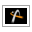AstroGrav Help /Data Elements /Other ElementsVersion 5.0.1

#Other Elements

An object's other elements are miscellaneous values that each provide a possible alternative to one of the orbital elements.

The other elements are as follows.

• Eccentric Longitude is the longitude of the eccentric position, and has the symbol F. This is an alternative way to describe the position of the object on its orbit, and is the sum of the peri longitude and the eccentric anomaly (see below). It is a technical quantity used to convert from the true longitude to the mean longitude, or vice-versa. See a textbook or website on spherical astronomy for further information.

The longitude of the eccentric position may be any number, but will be automatically normalized to lie in the interval [0, 2π) if the orbit is closed (a circle or an ellipse).

• Mean Longitude is the longitude of the mean position, and has the symbol L. This is an alternative way to describe the position of the object on its orbit, and is the sum of the peri longitude and the mean anomaly (see below). It has the useful property that it changes constantly with the passage of time. See a textbook or website on spherical astronomy for further information.

The longitude of the mean position may be any number, but will be automatically normalized to lie in the interval [0, 2π) if the orbit is closed (a circle or an ellipse).

• True Anomaly is the anomaly of the true position, and has the symbol ν (or TA in the orbital elements diagram). This is an alternative way to describe the position of the object on its orbit, and is the angle between the point on the orbit that is closest to the parent and the position of the object on its orbit.

The anomaly of the true position may be any number, but will be automatically normalized to lie in the interval (-π, π] if the orbit is closed (a circle or an ellipse), and will be automatically normalized to lie in the interval (-acos(-1/e), acos(-1/e)) if the orbit is open (a parabola or a hyperbola).

• Eccentric Anomaly is the anomaly of the eccentric position, and has the symbol E. This is an alternative way to describe the position of the object on its orbit, and is a technical quantity used to convert from the true anomaly to the mean anomaly, or vice-versa. See a textbook or website on spherical astronomy for further information.

The anomaly of the eccentric position may be any number, but will be automatically normalized to lie in the interval (-π, π] if the orbit is closed (a circle or an ellipse).

• Mean Anomaly is the anomaly of the mean position, and has the symbol M. This is an alternative way to describe the position of the object on its orbit, and has the useful property that it changes constantly with the passage of time. See a textbook or website on spherical astronomy for further information.

The anomaly of the mean position may be any number, but will be automatically normalized to lie in the interval (-π, π] if the orbit is closed (a circle or an ellipse).

• Peri Argument is the argument of the pericenter position, and has the symbol ω. This is the angle between the ascending node and the point on the orbit that is closest to the parent.

The argument of the pericenter position may be any number, but will be automatically normalized to lie in the interval [0, 2π).